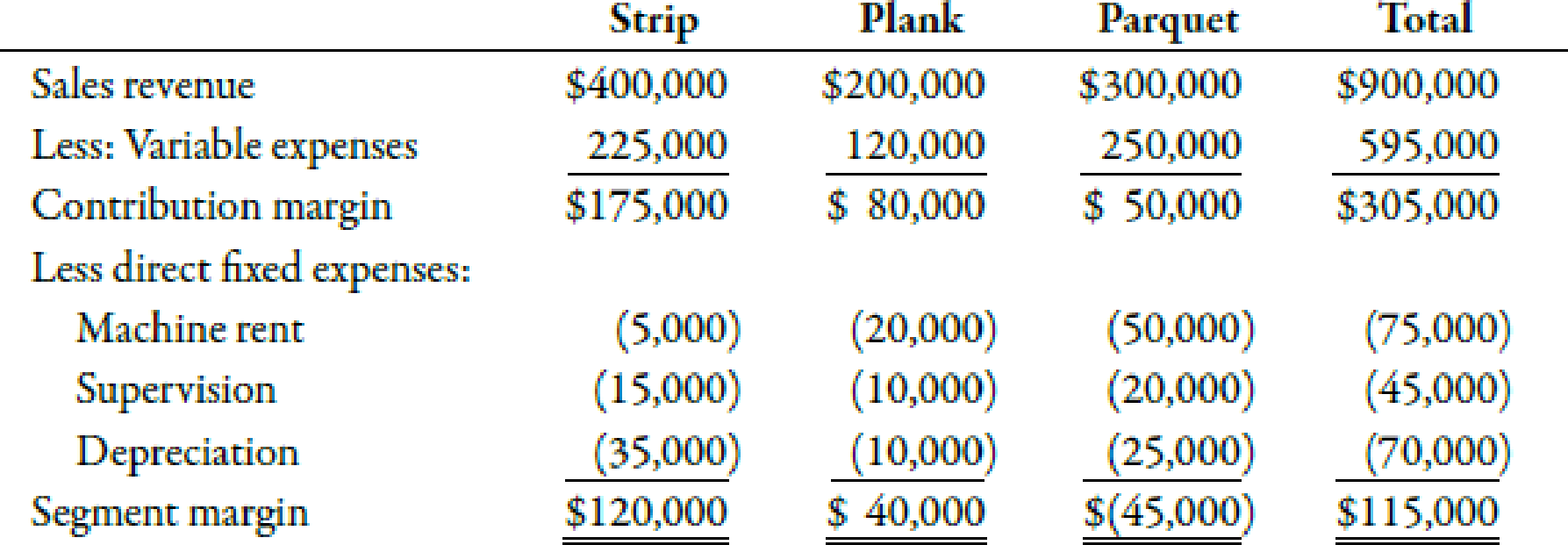Chapter 8, Problem 20BEA### Managerial Accounting: The Corners...

7th Edition
Maryanne M. Mowen + 2 others
ISBN: 9781337115773

#### Solutions

Chapter
Section### Managerial Accounting: The Corners...

7th Edition
Maryanne M. Mowen + 2 others
ISBN: 9781337115773
Textbook Problem
65 views

# Use the following information for Brief Exercises 8-19 and 8-20:Shown below is a segmented income statement for Hickory Company’s three wooden flooring product lines:8-20 Structuring a Keep-or-Drop Product-Line Problem with Complementary EffectsRefer to the information for Hickory Company on the previous page. Relevant fixed costs associated with this line include 80% of parquet’s machine rent and all of parquet’s supervision salaries. In addition, assume that dropping the parquet product line would reduce sales of the strip line by 10% and sales of the plank line by 5%. All other information remains the same.Required: 1. If the parquet product line is dropped, what is the contribution margin for the strip line? For the plank line? 2. Which alternative (keep or drop the parquet product line) is now more cost effective and by how much?

1.

To determine

Calculate the amount of contribution margin for strip and plank line if parquet is dropped.

Explanation

Contribution Margin:

Contribution margin can be defined as the amount of difference between sales revenue and the variable expense. It means the amount of sales left after covering variable expenses.

The amount of contribution margin for the strip line is $175,000. The reduction in the sale by 10% will result in a decrease in the amount of variable expense by 10%. This implies that the contribution margin will also get reduced by 10%. The contribution margin for the plank line is$80,000. Similarly, the contribution margin for the plank line will be reduced by 5%.

Use the following formula to calculate the reduced contribution margin if parquet is dropped:

Revised contribution margin=(Reduced contribution margin for strip line+Reduced contribution margin for plank line)

Substitute $157,500 for reduced contribution margin for strip line and$76,000 for reduced contribution margin for plank line in the above formula.

Revised contribution margin=$157,500+$76,000=$233,500 Therefore, the revised contribution margin when the parquet product is dropped is$233,500.

Working Notes:

1

2.

To determine

Evaluate the most effective alternative (keep or drop the parquet) and its amount.

### Still sussing out bartleby?

Check out a sample textbook solution.

See a sample solution

#### The Solution to Your Study Problems

Bartleby provides explanations to thousands of textbook problems written by our experts, many with advanced degrees!

Get Started

#### Find more solutions based on key concepts